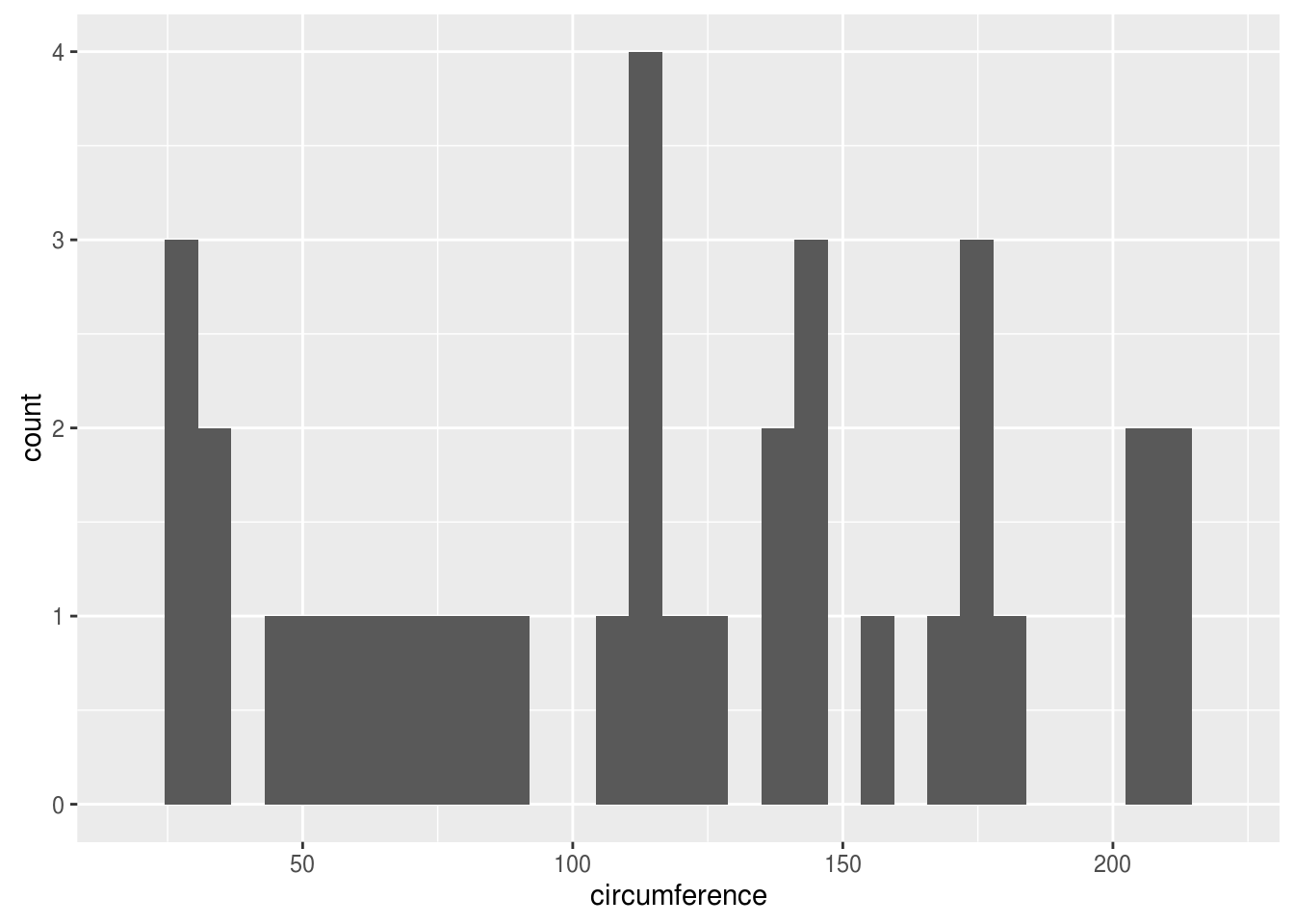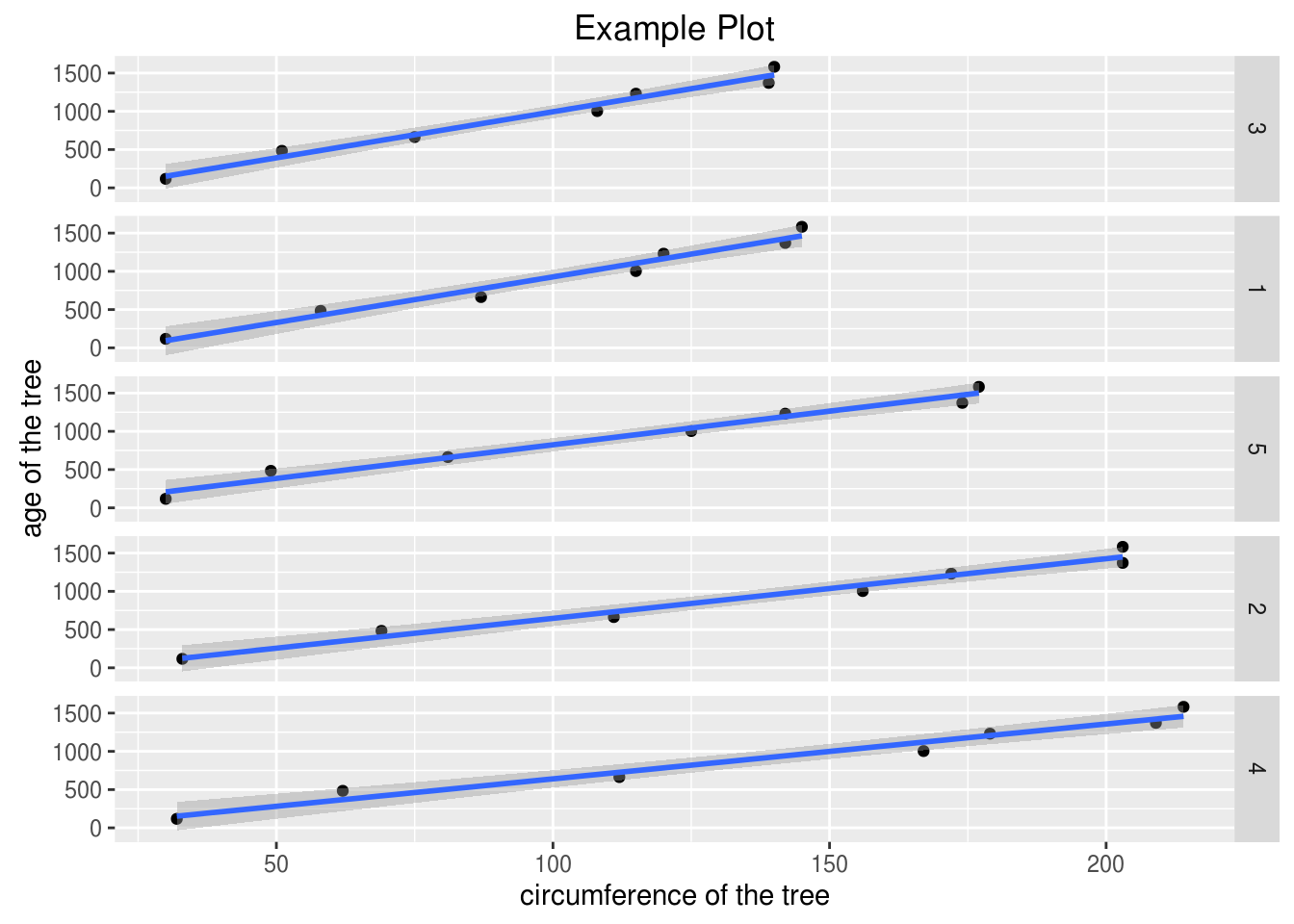# Intro to the Grammar of Graphics

In developing graphs, there are certain core principles that need to be addressed in order to provide a graph that communicates meaning clearly to an audience. Many of these core principles are addressed in the book “The Grammar of Graphics” by Leland Wilkinson.The concepts of Wilkinson’s book were used to create the “ggplot2” r package by Hadley Wickham. This post will explain some of the core principles needed in developing high-quality visualizations. In particular, we will look at the following.

• Aesthetic attributes
• Geometric objects
• Statistical transformations
• Scales
• Coordinates
• Faceting

One important point to mention is that when using ggplot2 not all of these concepts have to be addressed in the code as R will auto-select certain features if you do not specify them.

Aesthetic Attributes and Geometric Objects

Aesthetic attributes are about how the data is perceived. This generally involves arguments in the “ggplot” relating to the x/y coordinates as well as the actual data that is being used. Aesthetic attributes are mandatory information for making a graph.

Geometric objects determine what type of plot is generated. There are many different examples such as bar, point, boxplot, and histogram.

To use the “ggplot” function you must provide the aesthetic and geometric object information to generate a plot. Below is coding containing only this information.

``````library(ggplot2)
ggplot(Orange, aes(circumference))+geom_histogram()``````
``## `stat_bin()` using `bins = 30`. Pick better value with `binwidth`.``The code is broken down as follows ggplot (data, aesthetic attribute(x-axis data at least)+geometric object())

Statistical Transformation Statistical transformation involves combining the data in one way or the other to get a general sense of the data. Examples of statistical transformation include adding a smooth line, a regression line, or even binning the data for histograms. This feature is optional but can provide additional explanation of the data.

Below we are going to look at two variables on one plot. For this, we will need a different geometric object as we will use points instead of a histogram. We will also use a statistical transformation. In particular, the statistical transformation is regression line. The code is as follows

``ggplot(Orange, aes(circumference, age))+geom_point()+stat_smooth(method="lm")``The code is broken down as follows ggplot (data, aesthetic attribute(x-axis data at least)+geometric object()+ statistical transformation(type of transformation))

Scales Scales is a rather complicated feature. For simplicity, scales have to do with labeling the title, x and y-axis, creating a legend, as well as the coloring of data points. This use of this feature is optional.

Below is a simple example using the “labs” function in the plot we develop in the previous example.

``ggplot(Orange, aes(circumference,age))+geom_point()+stat_smooth(method="lm") +  labs(title="Example Plot", x="circumference of the tree", y="age of the tree")``The plot now has a title and clearly labeled x and y axises

Coordinates Coordinates is another complex feature. This feature allows for the adjusting of the mapping of the data. Two common mapping features are cartesian and polar. Cartesian is commonly used for plots in 2D while polar is often used for pie charts.

In the example below, we will use the same data but this time use a polar mapping approach. The plot doesn’t make much sense but is really just an example of using this feature. This feature is also optional.

``ggplot(Orange, aes(circumference, age))+geom_point()+stat_smooth(method="lm")+labs(title="Example Plot",x="circumference of the tree", y="age of the tree")+coord_polar()``The last feature is faceting. Faceting allows you to group data in subsets. This allows you to look at your data from the perspective of various subgroups in the sample population.

In the example below, we will look at the relationship between circumference and age by tree type.

``ggplot(Orange, aes(circumference, age))+geom_point()+stat_smooth(method="lm")+labs(title="Example Plot",x="circumference of the tree", y="age of the tree")+facet_grid(Tree~.)``Now we can see the relationship between the two variables based on the type of tree. One important thing to note about the “facet_grid” function is the use of the “.~” If this symbol “~.” is placed behind the categorical variable the charts will be stacked on top of each other is in the previous example.

However, if the symbol is written differently “.~” and placed in front of the categorical variable the plots will be placed next to each other as in the example below

``ggplot(Orange, aes(circumference, age))+geom_point()+stat_smooth(method="lm")+labs(title="Example Plot",x="circumference of the tree", y="age of the tree")+facet_grid(.~Tree)``Conclusion

This post provided an introduction to the grammar of graphics. In order to appreciate the art of data visualization, it requires understanding how the different principles of graphics work together to communicate information in a visual manner with an audience.